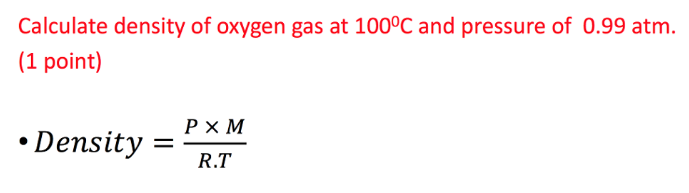# Problem: Calculate density of oxygen gas at 100°C and pressure of 0.99 atm. Density = P x M/R.T

###### FREE Expert Solution
87% (441 ratings)###### Problem Details

Calculate density of oxygen gas at 100°C and pressure of 0.99 atm.

Density = P x M/R.T